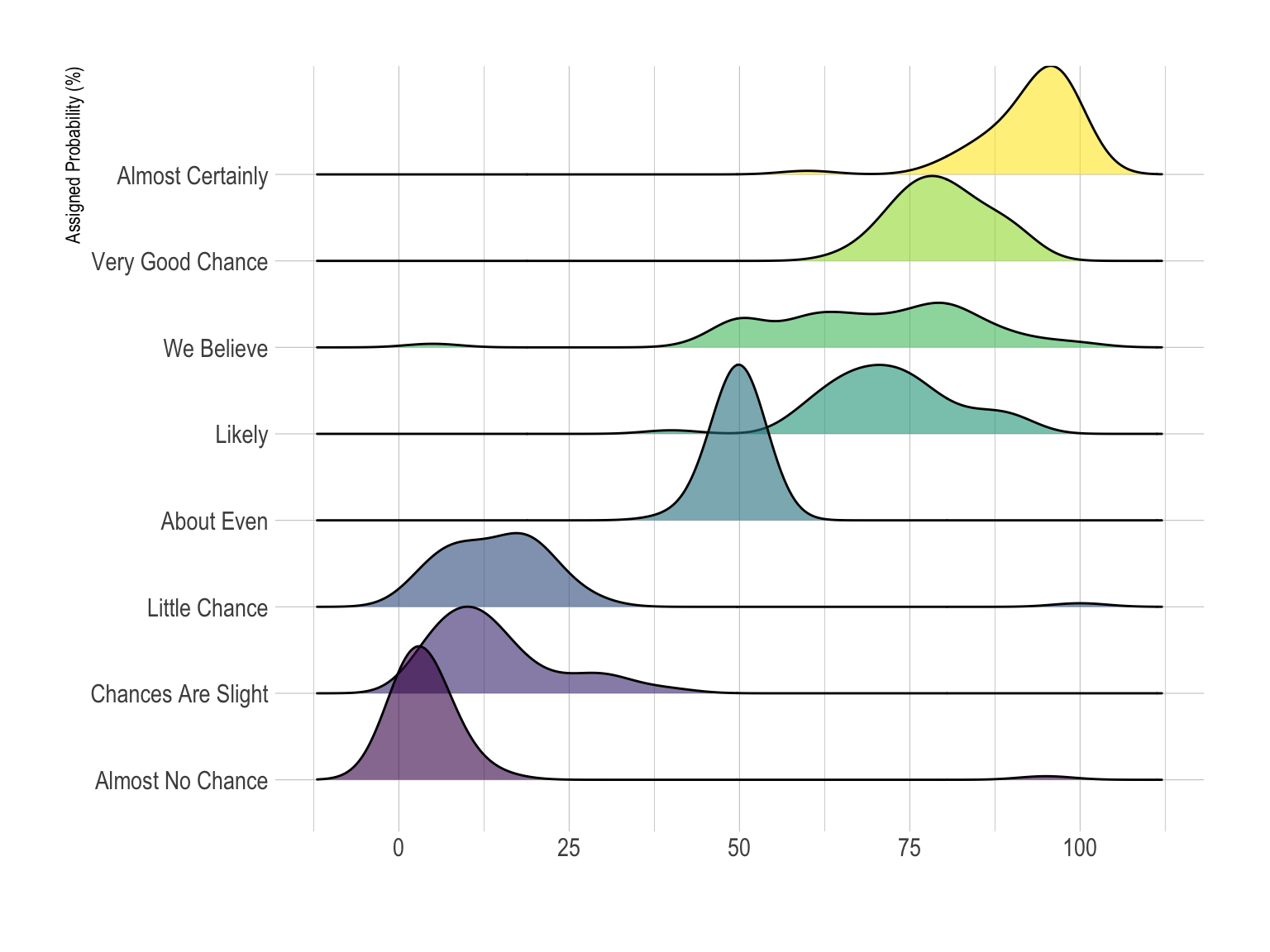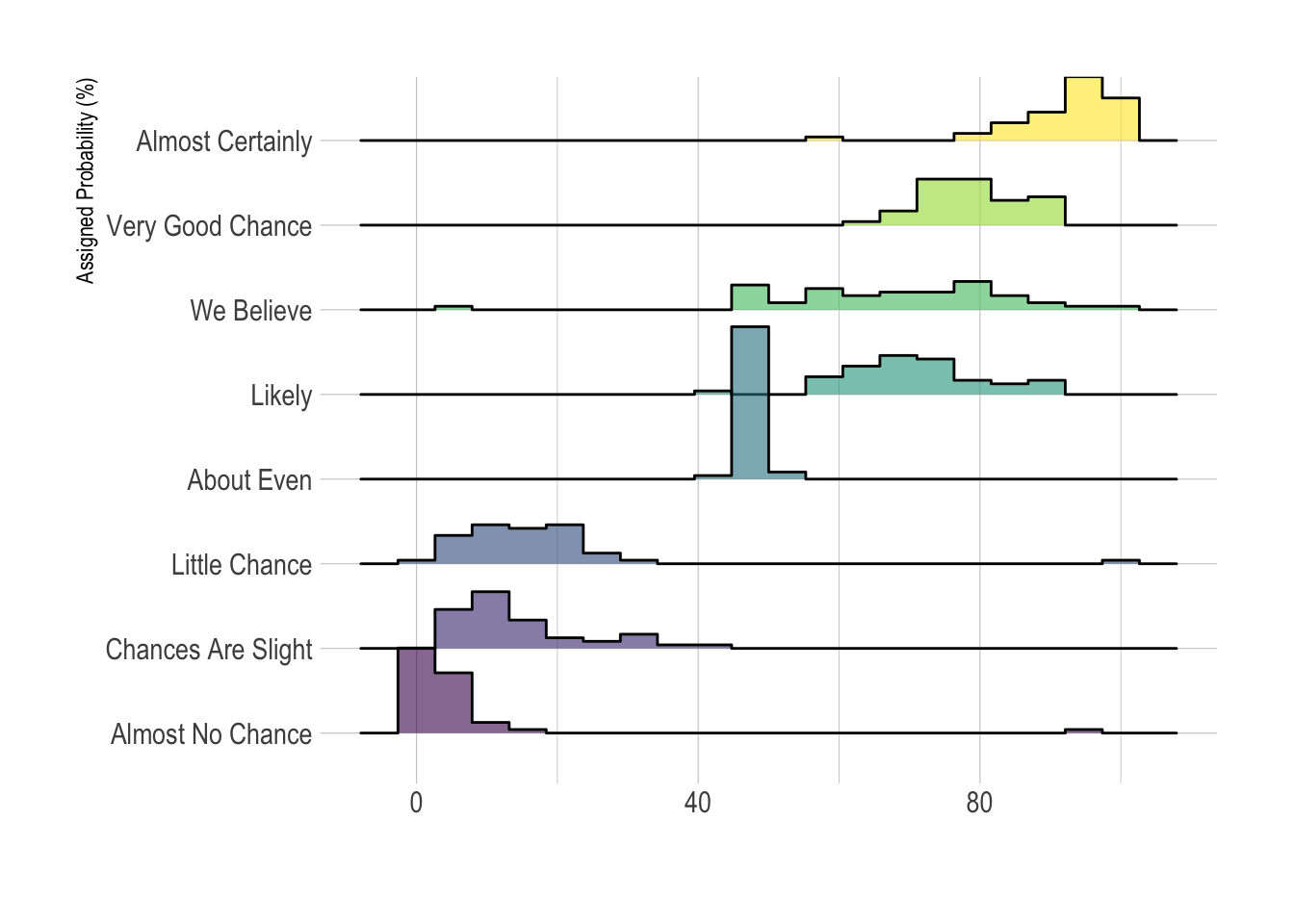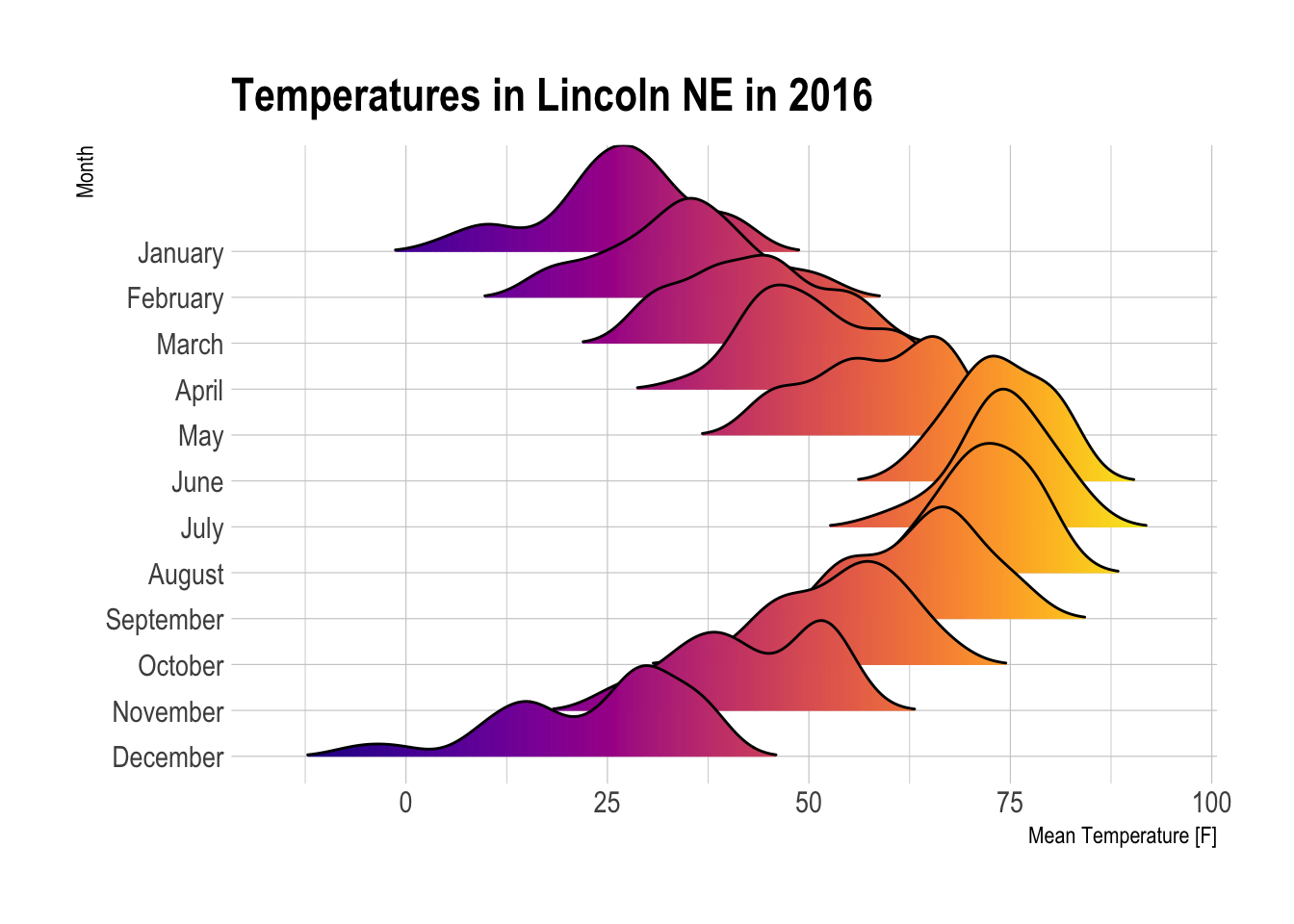# Definition

A Ridgeline plot (sometimes called Joyplot) shows the distribution of a numeric value for several groups. Distribution can be represented using histograms or density plots, all aligned to the same horizontal scale and presented with a slight overlap.

Here is an example showing how people perceive probability. On the /r/samplesize thread of reddit, questions like What probability would you assign to the phrase “Highly likely” were asked. Answers between 0 and 100 were recorded, and here is the distribution for each question:

``````# Libraries
library(tidyverse)
library(hrbrthemes)
library(viridis)

data <- data %>%
gather(key="text", value="value") %>%
mutate(text = gsub("\\.", " ",text)) %>%
mutate(value = round(as.numeric(value),0)) %>%
filter(text %in% c("Almost Certainly","Very Good Chance","We Believe","Likely","About Even", "Little Chance", "Chances Are Slight", "Almost No Chance"))

library(ggridges)

data %>%
mutate(text = fct_reorder(text, value)) %>%
ggplot( aes(y=text, x=value,  fill=text)) +
geom_density_ridges(alpha=0.6, bandwidth=4) +
scale_fill_viridis(discrete=TRUE) +
scale_color_viridis(discrete=TRUE) +
theme_ipsum() +
theme(
legend.position="none",
panel.spacing = unit(0.1, "lines"),
strip.text.x = element_text(size = 8)
) +
xlab("") +
ylab("Assigned Probability (%)")``````Disclaimer: This idea originally comes from a publication of the CIA which resulted in this figure. Then, Zoni Nation cleaned the reddit dataset and built graphics with R.

# What for

• Ridgeline plots make sense when the number of group to represent is `medium to high`, and thus a classic window separation would take to much space. Indeed, the fact that groups overlap each other allows to use space more efficiently. If you have less than ~6 groups, dealing with other distribution plots is probably better.

• It works well when there is a clear pattern in the result, like if there is an obvious ranking in groups. Otherwise group will tend to overlap each other, leading to a messy plot not providing any insight.

# Variation

• The above example is a ridgeline plot using a set of density plots. It is possible to use histograms as well:
``````data %>%
mutate(text = fct_reorder(text, value)) %>%
ggplot( aes(y=text, x=value,  fill=text)) +
geom_density_ridges(alpha=0.6, stat="binline", bins=20) +
scale_fill_viridis(discrete=TRUE) +
scale_color_viridis(discrete=TRUE) +
theme_ipsum() +
theme(
legend.position="none",
panel.spacing = unit(0.1, "lines"),
strip.text.x = element_text(size = 8)
) +
xlab("") +
ylab("Assigned Probability (%)")````````````ggplot(lincoln_weather, aes(x = `Mean Temperature [F]`, y = `Month`, fill = ..x..)) +
geom_density_ridges_gradient(scale = 3, rel_min_height = 0.01) +
scale_fill_viridis(name = "Temp. [F]", option = "C") +
labs(title = 'Temperatures in Lincoln NE in 2016') +
theme_ipsum() +
theme(
legend.position="none",
panel.spacing = unit(0.1, "lines"),
strip.text.x = element_text(size = 8)
) ``````# Related

The R and Python graph galleries are 2 websites providing hundreds of chart example, always providing the reproducible code. Click the button below to see how to build the chart you need with your favorite programing language.